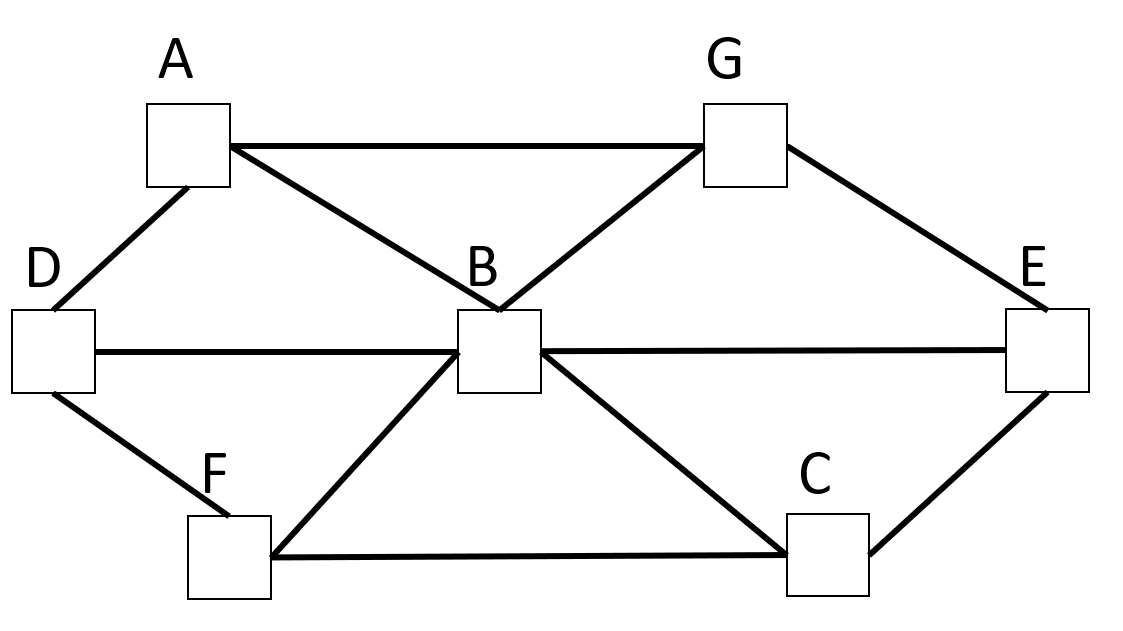# Number Pattern

Directions: Using the digits 1 to 7, at most one time each, place a digit in each circle so that the sum of the numbers in 3 squares (the middle horizontal line or 2 diagonals) are same.

e.g A+B+C or D+B+E or F+B+G

Is there more than one solution?### Hint

Be strategic to think about the number in the center circle.

5 2
7 4 1
6 3

Source: Al Oz

## Equivalent Expressions with Fractions

Directions: Using the digits 0 to 9 at most one time each and choosing either …

1.a=7 b=1 c=2
d=6 e=3
f=5 g=4

•OMG I didn’t relize that!

2.A=2 B=7 C=5
D=3. E=4
F=1. G=6

or change the pairing to get similar solutions